# Revision Notes: Nuclear Physics Notes | Study Mock Test Series for JEE Main & Advanced 2022 - JEE

## JEE: Revision Notes: Nuclear Physics Notes | Study Mock Test Series for JEE Main & Advanced 2022 - JEE

The document Revision Notes: Nuclear Physics Notes | Study Mock Test Series for JEE Main & Advanced 2022 - JEE is a part of the JEE Course Mock Test Series for JEE Main & Advanced 2022.
All you need of JEE at this link: JEE
• Nuclear Physics:- Branch of physics dealing with the study of nucleus is called nuclear Physics.
• Constituents of nucleus (Nucleons) :-
(a) Protons:-
Mass of proton, mp = 1.6726×10-27 kg
Charge of proton = 1.602×10-19 C
(b) Neutron:-
Mass of neutron, m= 1.6749×10-27 kg
(c) 1 atomic mass unit (1 amu) = 1.66×10-27 kg
(d) 1 amu = 1 u = 931.5 MeV
• Properties of nucleus:-
(a) Charge on nucleus = 1.602×10-19 Z coulomb
(b) Size of nucleus:-
The radius r of the nucleus depends upon the atomic mass A of the element.
r =R0A1/3
Here R0 = 1.2×10-13 cm     (1 Fermi =10-13 cm)
Volume of the nucleus:-
V = 4/3 πr= 4/3 π (R0A1/3)3
(c) Density of nucleus:-
Density of nucleus = mass/volume = A/(4/3 πR03A) = [3/(4πR03)]
• Isotopes:- Nuclei having same atomic number Z but different mass number A are called isotopes.
• Isobars:- Nuclei having same mass number A but different atomic number Z are called isobars.
• Isotones:- Nuclei having the same number of neutrons (N) but different atomic number (Z) are called isotones.
• Nature of nuclear force:-
(a) Nuclear forces are attractive in nature.
(b) Nuclear forces are charge independent.
(c) These are short range forces.
(d) Nuclear forces decrease very quickly with distance between two nucleons.
(e) Nuclear forces are spin dependent.
• Mas defect:- If the mass of the nucleus HZA is M, then the mass defect,
ΔM = [Zmp + (A-Z)mn – M]?
Here, mp and mn are the masses of the proton and neutron respectively.
• Binding Energy:-
Binding Energy = (ΔM) c2 = [Zmp + (A-Z)mn – M] c2
If we use atomic mass instead of nuclear masses, then,
Binding Energy, B = [ZmH + (A-Z)mn – Mat] c2
Here Mat is the mass of the atom  and mH is the mass of the hydrogen atom.
• Binding energy per nucleon:-
B/A = [Zm+ (A-Z)mn – M] c2 /A
If we use atomic mass instead of nuclear masses, then,
B/A = [ZmH + (A-Z)m– Mat] c2/A
• Radioactivity:- The phenomenon by virtue of which substance, spontaneously, disintegrate by emitting certain radiations is called radioactivity.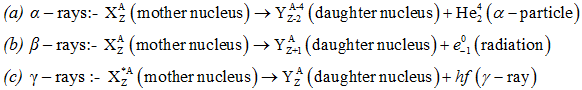• Alpha decay:- It is the process in which a parent nucleus decays into the daughter nucleus by ejecting an alpha particle.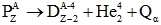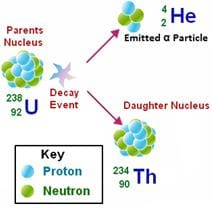• Beta decay:- It is the process in which a parent nucleus decays into the daughter nucleus by ejecting an electron.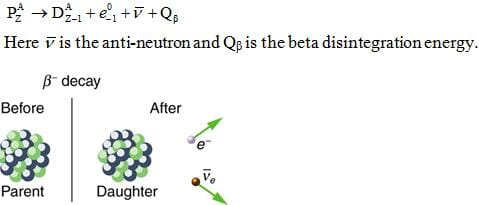• Gama decay:- Sometimes the daughter nucleus is left in the excited state. It decays in to any other lower state or ground state by emitting γ-rays.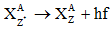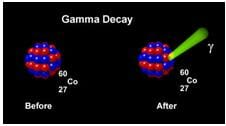• Laws of radioactivity:-(a) Radioactivity is due to the disintegration of a nucleus.
(b) Rate of disintegration is not affected by the external conditions like temperature and pressure etc.
(c) Law of conservation of charge holds good in radioactivity.
(d) The disintegration is accompanied by the emission of energy in terms of α, β and γ-rays either single or all at a time.
Emission of α- particle results in a decrease in its atomic number by 2 and a decrease in its atomic weight by 4.
Emission of β- particle results in an increase in its atomic number by 1 while its atomic weight remains unaffected.
Emission of γ- rays results neither in a change of atomic number nor in a change of atomic weight.
(e) Each of the product disintegration is a new element having physical and chemical properties different from those of the parent atom.
(f) Rate of disintegration of the radioactive substance, at any instant, is directly proportional to the number of atoms present at that instant.
N = N0e-λt
If, t = 1/λ, then, N = N0/e
Therefore, radioactive decay constant is defined as the reciprocal of time in which the number of atoms of radioactive sample is reduced to N0/e.
• Half-life (T1/2):- Half-life of a radioactive substance is defined as the time during which the number of atoms of the substance are reduced to half their original value.
T1/2 = 0.693/λ
Thus, half-life of a radioactive substance is inversely proportional to its radioactive decay constant.
• Average life (Tav):- Arithmetic mean of the lives of all the atoms is known as mean life or average life of the radioactive substance.
Tav = sum of lives of all atoms / total number of atoms
Average life of a radioactive substance is equal to the reciprocal of its radioactive decay constant.
• Relation between T1/2 and Tav:-
T1/2 = 0.693× Tav
Half-life = 0.693×average life
(a) Curie (Ci):- Radioactivity of a substance is said to be one curie if its atoms disintegrate at the rate of 3.7×1010 disintegrations per second.
(b) Rutherford (rd):- Radioactivity of a substance is said to be 1 Rutherford if its atoms disintegrate at the rate of 10disintegrations per second.
• Relation between Curie and Rutherford:-
1 C = 3.7×104 rd
• Nuclear fission:- Nuclear fission is the process by  which a nucleus breaks up in such a way that the two products obtained are of comparable sizes.
Fission of U92235 by fast moving neutrons is represented as,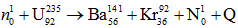Value of Q in this reaction is 200.4443 MeV.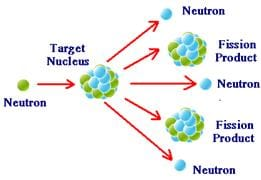• Chain reaction:-The above quoted fission reaction proceeding in an uncontrolled manner is known as chain reaction and forms the basis of atom bomb. Following conditions must be met with, for the chain reaction to proceed.
(a) The fissionable material should be larger than a certain minimum size.
(b) The material should be highly pure.
• Nuclear reaction:- Fission reaction of  U92235can be made to proceed in a controlled manner by slowing down the three emitted neutrons. This is achieved by passing them through moderators. Heavy water and carbon rods can be used as moderators. A device in which the fission proceeds in a controlled manner is called a nuclear reaction.
• Nuclear fusion:- A fusion reaction is one in which two lighter nuclei get fused together to form a heavy nucleus. It is a highly exothermic reaction and produces energy, on a scale, much higher than that produced in fission.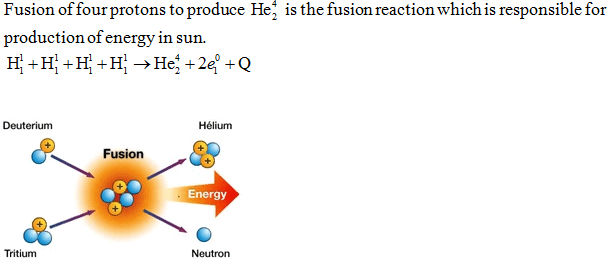• Decay constant for two channels:-
(a) λ= λ1+ λ2
(b) T = T1T2/T1+T2
• Gamma intensity absorption:-
I = I0e - μx
• Laws governing nuclear reaction:-
(a) Law of conservation of charge
(b) Law of conservation of number of nucleons
(c) Law of conservation of energy.
• Classification of nuclear reaction:- Nuclear reaction can be classified into following categories.
(a) Elastic scattering
(b) Inelastic scattering
(c) Simple capture
(d) Disintegration
• Q-value of nuclear reaction:-
Q= {(mi+mt) – (mp+m0)} c2
• Radioisotope:- An isotope, obtained in process of artificial transmutation, which exhibits radioactivity is called a radio-isotope. It can be put to following uses:
(a) Tracer
(b) In medicine
(c) In industry
The document Revision Notes: Nuclear Physics Notes | Study Mock Test Series for JEE Main & Advanced 2022 - JEE is a part of the JEE Course Mock Test Series for JEE Main & Advanced 2022.
All you need of JEE at this link: JEEUse Code STAYHOME200 and get INR 200 additional OFF

## Mock Test Series for JEE Main & Advanced 2022

2 videos|258 docs|160 tests

Track your progress, build streaks, highlight & save important lessons and more!

,

,

,

,

,

,

,

,

,

,

,

,

,

,

,

,

,

,

,

,

,

;